## 2.3.5 Monte Carlo methods

The Monte Carlo Metropolis method has been widely used to obtain the equilibrium properties of a physical system in contact with a heat bath of temperature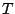[Binder 97]. Ising models with Monte Carlo constitute an ideal system from both learning and modeling point of view. In localized moments approximation the method has been used in conjunction with Heisenberg models [Hinzke 98] and antiferromagnetism is studied with the same tools [Acharyya 00]. The method makes changes in the configuration and accept or reject the new configuration according to some probability based on the Boltzmann statistics. One iteration of this algorithm is called a Monte Carlo (MC) step. The method is known as Metropolis Monte Carlo method. The Metropolis Monte Carlo simulations generate configurations with the system variables values according to the Boltzmann distribution, obtaining the equilibrium properties from the average of the configurations. However, the Monte Carlo method is also a viable method to obtain nonequilibrium properties. A classical example is the random walk of a Newtonian particle with thermal fluctuations. In magnetism the Metropolis method has been used to study the thermally induced magnetic collective magnetization relaxation [González 95]. The dynamics of magnetic systems with MC method do not consider the precession of the magnetic moment. The method will accept a configuration that decreases the energy but the moment will not describe the correct precession. Accordingly, the MC method will fail to describe the dynamics in the situations in which the precession is important and will succeed in case where it is not. This way we can expect good results of the method in cases of high temperature or large damping, where the diffusion prevails over the precession. Moreover, the MC step has not an associated timestep. To solve this problem the Time Quantified Metropolis Monte Carlo (TQMC) algorithm [Nowak 00,Chubykalo 03a] has been proposed. The main relation of TQMC is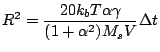(2.61)

where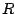is the radius of the cone around the current moment position inside which the trial moment lays andis the equivalent timestep of the MC step. This timestep has to be chosen less than the characteristic time scale of the relaxation. From Eq. (2.61) it is clear that the advantage of this method is the possibility to increase the timestep with smaller temperatures that need a longer simulations in LD simulations. However, the associated acceleration is linearly proportional to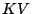, while an exponential acceleration is desirable, according to the Arrhenius-Néel law (2.58). More recently, X. Cheng et al. [Cheng 06] have reported a similar method that preserves the precession of the magnetic moment. To calibrate the timestep of the Monte Carlo step X. Cheng used the Fokker-Planck equation instead of a direct mapping to the Langevin dynamics. Nevertheless, if the magnetization precession has to be taken into account, the timestep associated with MC in this case is of the order of the timestep for the LD simulations.

A more successful method to calculate long time thermal magnetization dynamics is the Kinetic Monte Carlo method known in magnetism sometimes as Charap method [Kanai 91]. The method evaluates the rate of all the reversal modes, which link the minimum where the magnetization is presently located with other minimum in the system, and chooses the mode according to the probability:(2.62)

where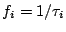is the rate and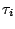is the time constant for the reversal mode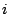. The method requires the evaluation of the saddle point and the energy barrier for each reversal mode. The method originally proposed by Charap calculated the switching rates of the reversal of the individual moments. This approach neglects the possible collective reversal modes appearing in strongly interacting systems. This was overcome by O. Chubykalo et al. [Chubykalo-Fesenko 05], who suggested an algorithm based on the combination of Langevin dynamics and Metropolis Monte Carlo in order to explore the possible reversal modes and used it to evaluate the stability of granular longitudinal magnetic recording media. Due to the exponential nature of the Arrhenius-Néel law used to calculate the switching rates, this method can predict the thermal relaxation in years scales.

2008-04-04